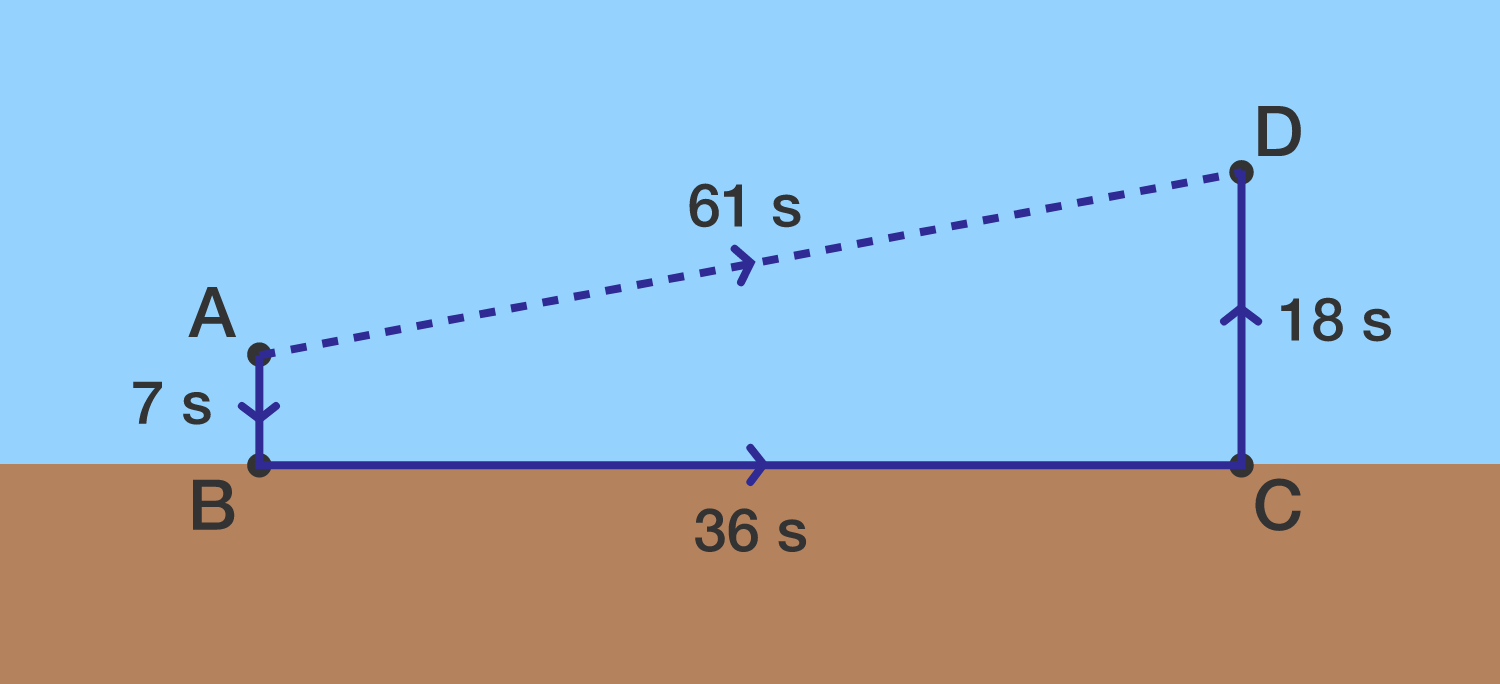# Asap

Geometry Level 2

Agatha is at point $A,$ and needs to reach her son Damien at point $D$ as soon as possible. She can swim at a constant rate, and she can also run along the shore at a constant rate (her swimming and running speeds are different).

The diagram shows how long each segment takes her to travel. A direct route $A \rightarrow D$ takes 61 s, but so does a route of $A \rightarrow B \rightarrow C \rightarrow D.$

Find the minimum possible amount of time (in seconds) required for Agatha to reach Damien.Segments $\overline{AB}$ and $\overline{CD}$ are perpendicular to the shoreline.

Note: When Agatha swims to and from the shoreline, the angles don't have to be 90 degrees.

×